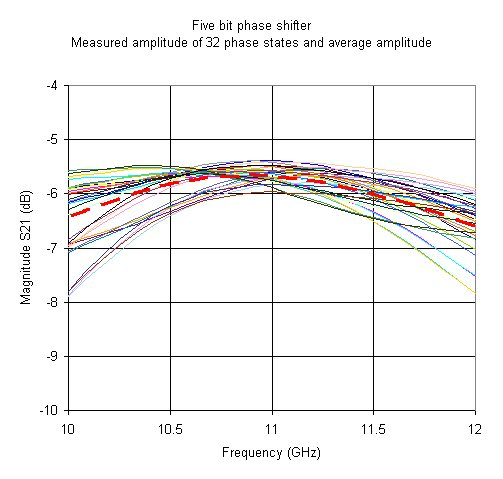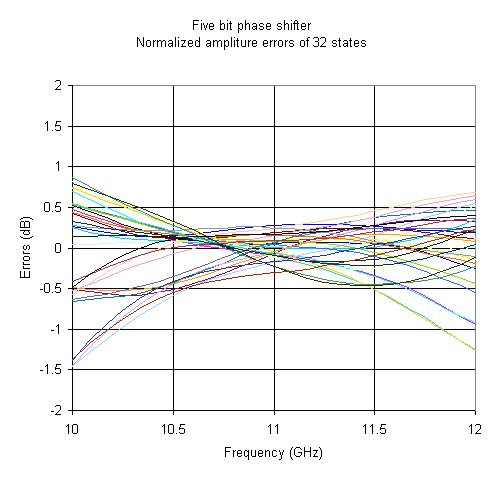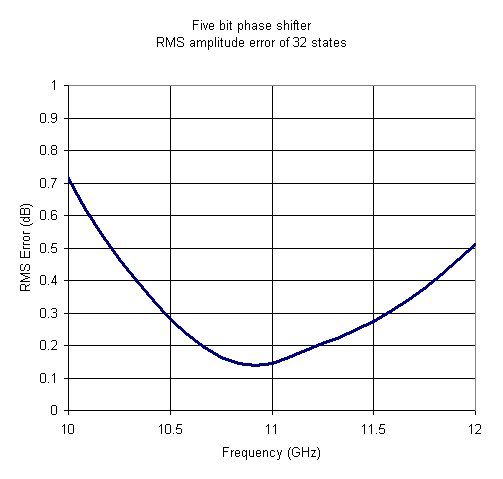# Phase Shifter RMS Amplitude Error

New for January 2007! Here we'll present the RMS amplitude error calculation for a phase shifter in plain English! The main application for digital phase shifters and attenuators is in phased array systems.

An N-bit digital phase shifter has 2^N phase states. In almost most applications we are trying to control phase over 360 degrees. Therefore a two-bit phase shifter will have four phase states:

0, 90, 180, 270

A three bit phase shifter has eight phase states:

0, 45, 90, 135, 180, 225, 270, 315

A four bit phase shifter has 16 phase states:

0, 22.5, 45, 67.5, 90, 112.5, 135, 157.5, 180, 202.5, 225, 247.5, 270, 292.5, 315, 337.5

And so on.

We've seen designs that go up to eight bits, but typically you will see digital phase shifters with three, four or five bits.

Calculating RMS amplitude error can be broken down into four easy steps:

1. Gather amplitude data in dB
2. Compute average amplitude in dB
3. Compute amplitude errors of all states
4. Perform root-mean-square of amplitude errors

Let's give an example of a five-bit phase shifter. We faked some phase data for thirty-two phase states and put it into Excel. It's not like TriQuint or M/A-COM supplies full data on their phase shifter products! The plot below shows amplitudes of 32 phase states. The dashed red line is the average amplitude, which is calculated by summing the amplitudes at each frequency and dividing by 32.Next we subtract the average amplitude from each state. Note how the values are centered around zero dB.We'll combine the last two steps in this final graph. Here we've summed the squares of each error, divided by 32 and taken the square root:Pretty simple stuff, but it isn't presented anywhere else on the world wide web! By the way, an amplitude error of 0.5 dB over the intended band is a pretty good result.

Author : Unknown Editor